sketch in CATIA | 12CAD.com

# Constraints used for a sketch in CATIA

Only a precise 2D geometry will create a precise 3D component.

To create a precise 2D sketch in CATIA, we use constraints. What  does “constraint” actually means?

It means that we are restricting the degree of freedom of the sketch. Consider, you want to constraint a line. If you provide its length, then it ensures that the length of the line is under control.

Similarly, providing location of the start and end point of line will make it fully constrained

i.e. the line will not move anywhere until you change the dimensions.

Like in most of the parametric CAD software, CATIA provides two types of constraints.

When you give numerical value to a geometry, it means you have given Dimensional constraint. It may be Length or Angle.

As shown in the figure below, the start point of the line is at (0, 0) and the end point is automatically described by length and angle.

This makes the line fully constrained.Geometrical constraints include Parallelism, Symmetry, Coincidence, Tangency, Midpoint, Perpendicular, Concentricity, etc.

Geometrical constraints are usually given automatically by the software, but at some place we may need to give a particular constraint.

We will see how to use these constraints for a sketch.

In the Constraints toolbar you will find options like Constraint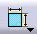and

Constraints Defined in Dialog Box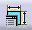Mostly, Constraint is used to give dimensions and Constraints Defined in Dialog Box is used to give geometrical constraints.

Constraints Defined in Dialog Box option will not be active unless you click on a geometry.

### Vertical/Horizontal

If you want a non horizontal/vertical line previously drawn to be wither horizontal or vertical, you can use the following technique.

1. Select the line.
2. Click on Constraints Defined in Dialog Box. A dialog box will appear.
3. Select Vertical or Horizontal, whichever is required.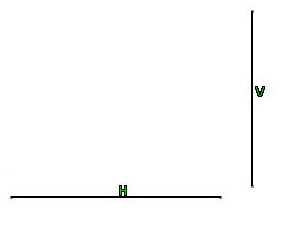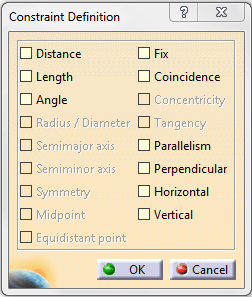### Parallelism

It makes two lines or a line with an axis parallel.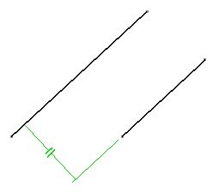How to give parallelism between two lines:

• Draw two lines. These should not be parallel. (While drawing these lines, CATIA will try making the lines parallel, but avoid that for the sake of experiment)
• Now select both lines with the help of Ctrl button. The Constraints Defined in Dialog Box will become active.
• Click on the check box provided for parallelism and click OK. The lines will be parallel now.

### Symmetry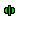This constraint allows us to draw symmetrical sketch about a center line. Let us see how to give symmetry.

• If you want two lines to be symmetric to each other, select those lines as well as select the center line.
• Select the check box provided for Symmetry.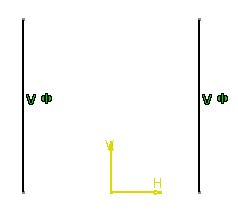One thing you should keep in mind while giving symmetry is the selection sequence of lines and center line, the order of selection affects the result.

You should always select the two lines first, and last the center line. Otherwise you will get a different result than required.

Now you must have noticed that for different selections, the Constraints Defined in Dialog Box activates different options. Similarly, if you select a circle (or arc) and a line (or another circle, arc, etc), it will activate Tangency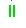If two circles are selected it will activate Concentricity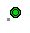These technique do nothing but help you get work done faster and help have better accuracy while working.